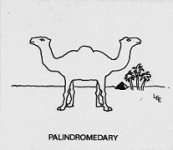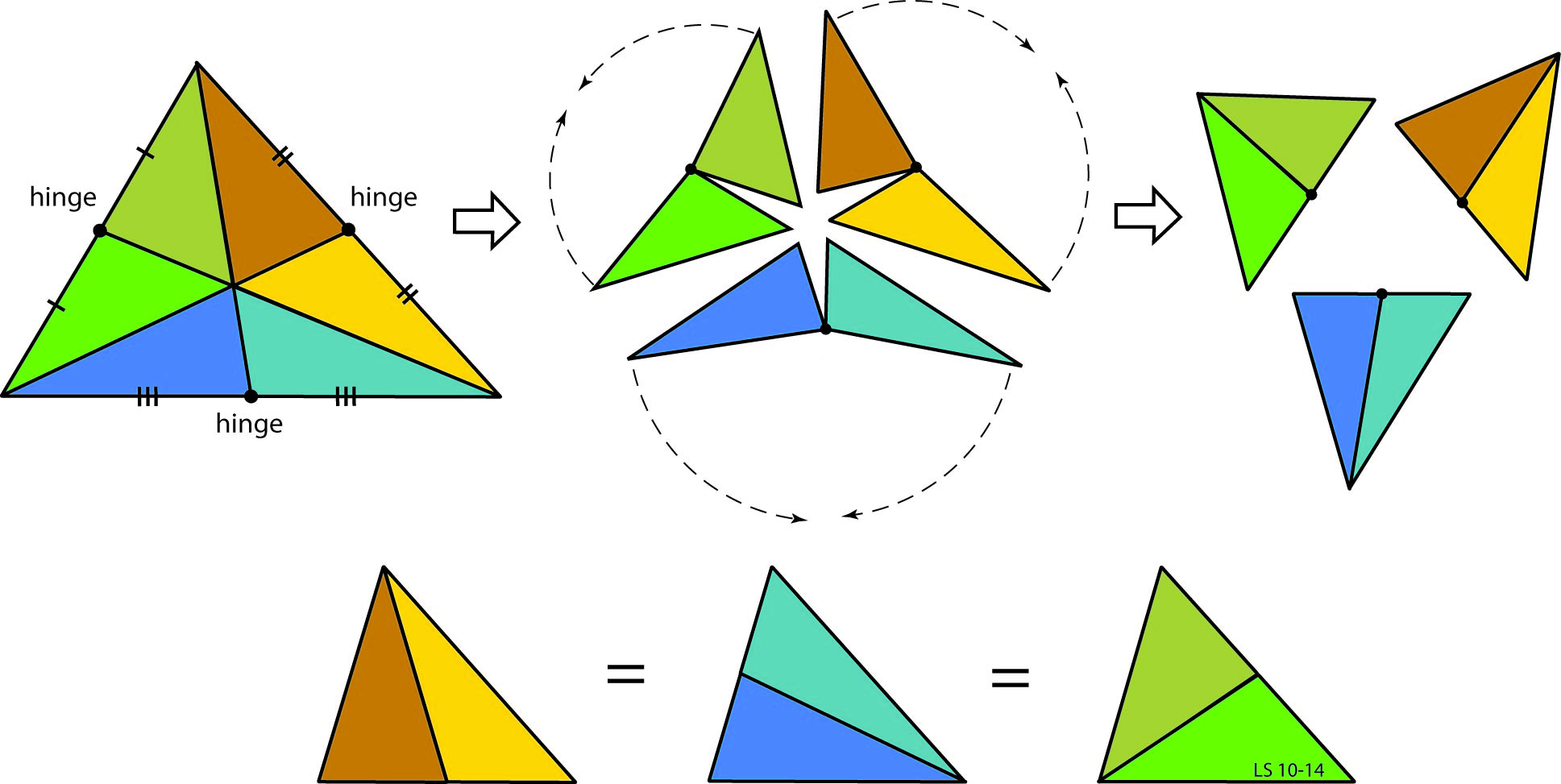# Lee Sallows

Home > Varia > Triangle Theorem

Triangle Theorem

The medians of any triangle dissect it into 6 smaller triangles, three adjacent pairs of which
share a common vertex point that is the midpoint of a side. If now the two triangles in each
pair are rotated about their common vertex, the one clockwise, the other anticlockwise, until
they meet so as to share a common side, then the three new triangles formed by the union of
each pair are congruent.Last Updated:
18-8-2016